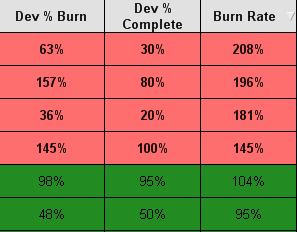# QlikView App Dev

Discussion Board for collaboration related to QlikView App Development.

Announcements
cancel
Showing results for
Did you mean:Not applicable

## Using a button to extract a variable's values

Hi,

I am making a straight table chart in Qlikview and I am trying to select certain values in the chart by using a button.

My chart looks like the image below. Burn rate comes from the variable 'vBurnRate' which comes from dividing Dev % Burn by Dev % Complete. It is currently \$(vBurnRate) in the expression. The colors are indicated by greater than or less than percentages. For example red is if the Burn Rate is <85% and also if it is >115%. It is yellow if it is 85-89% and also if it is 111-115%. It is green if it is 90-110%.I am trying to make a button that can select, based on Burn Rate, if it is red, yellow, or green based on the percentage range above.

10 RepliesMVPNot applicable
Author

The dimension I am using is the Dev % Complete which is just a number as a percent. For example 30%.

My expressions are:

- Dev % Burn:

\$(vDevBurn)

This variable's value is: (sum({\$<[Tracker.Category]={"Development"}>} [Tracker.Time Reported Actual]) / ([Developer.Dev Estimated Efforts]))

-Burn Rate:

\$(vBurnRate)

This variable's value is: \$(vDevBurn)/[Developer.Dev % Complete]

The background color expression for the fields are:

if(\$(vBurnRate)<'85%',rgb(255,110,110),

if(\$(vBurnRate)>='85%' and \$(vBurnRate)<'90%',rgb(255,255,0),

if(\$(vBurnRate)>='90%' and \$(vBurnRate)<='100%',rgb(34,139,34),

if(\$(vBurnRate)>='101%' and \$(vBurnRate)<='110%',rgb(34,139,34),

if(\$(vBurnRate)>='111%' and \$(vBurnRate)<='115%',rgb(255,255,0),

If(\$(vBurnRate)>'115%',rgb(255,110,110)

))))))MVP

Can you try this in a list box expression:

Aggr(If(\$(vBurnRate) < '85%', 'Red',

If(\$(vBurnRate)>='85%' and \$(vBurnRate)<'90%', 'Yellow',

If(\$(vBurnRate)>='90%' and \$(vBurnRate)<='100%', 'Green',

If(\$(vBurnRate)>='101%' and \$(vBurnRate)<='110%', 'Green',

If(\$(vBurnRate)>='111%' and \$(vBurnRate)<='115%', 'Yellow',

If(\$(vBurnRate)>'115%', 'Red')))))), [Dev % Complete])Master III

Hi,

Can you check the variable(vBurnRate) value shows with % or only numbers ? like 85% or 0.85?

may be try this..

if(\$(vBurnRate)<0.85,rgb(255,110,110),
if(\$(vBurnRate)>=0.85 and \$(vBurnRate)<0.9,rgb(255,255,0),
if(\$(vBurnRate)>=0.9 and \$(vBurnRate)<=1,rgb(34,139,34),
if(\$(vBurnRate)>=1.01 and \$(vBurnRate)<=1.1,rgb(34,139,34),
if(\$(vBurnRate)>=1.11 and \$(vBurnRate)<=1.15,rgb(255,255,0),
If(\$(vBurnRate)>1.15,rgb(255,110,110)
))))))Not applicable
Author

I cannot use a list box because I have two other fields that are in my chart. I have names of developers and a WR # they are working on. When I did try it, only a few numbers connected to the colors.Not applicable
Author

It works with both % and with numbers. When I tried <85% it worked and when I tried <0.85 it worked as well.Partner

Hi,

You can try this logic,

PFA.

Warm Regards,
SusovanMVP & LuminaryNot applicable
Author

That did not work.